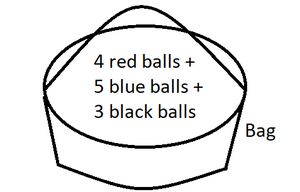# What is the probability of getting a non red ball from a bag containing 4 Red 5 blue and 3 black balls?

• Last Updated : 24 Jan, 2022

Probability is the likelihood of occurrence of the desired outcome(result) over the set of all possible outcomes (results) of a situation. Probability in simple terms indicates possibility. The probability of occurrence of an event denotes the ratio of the desired result to all possible results.

### Terms in probability

There are different terms often used in probability. Terms like an experiment, trial, event, mutually exclusive events, outcome, favorable event, etc. Let’s take a look at them in detail,

• Experiment: Experiment refers to any kind of activity with non-defined results.
• Trial: Trial refers to the different attempts of an experiment
• Event: Event refers to a trial with a well-defined outcome
• Mutually Exclusive Events: Mutually Exclusive Events are events that have nothing in common, and the occurrence of one event prevents the occurrence of another.
• Outcome: Outcome refers to the final result of trial
• Favorable Event: Favorable Events are the events with the desired outcome/result

Probability of occurrence of any event

The formal definition of the probability of occurrence of any event would be, Probability of occurrence of Favorable Event/ Probability of Occurrence of All Events (Favorable + Unfavorable). The total set of events in an event of probability is the sum of Favorable Events and Unfavorable Events. These tend to be the denominator of the Probability calculation ratio

### The probability of getting a non red ball from a bag containing 4 Red 5 blue and 3 black balls

Solution:figure 1: Bag containing the balls

Number of red balls in the bag = 4

Number of blue balls in the bag = 5

Number of black balls in the bag = 3

Total balls in the bag = 4 + 5 + 3 = 12

Probability of getting a red ball

= Red Balls in the bag/ Total number of Balls in the bag

= 4/12

Probability of getting a blue ball

= Blue Balls in the bag/ Total number of Balls in the bag

= 5/12

Probability of getting a black ball

= Black Balls in the bag/ Total number of Balls in the bag

= 3/12

Now, the Probability of getting a non-red ball can be calculated by three ways:

1st Way:

Probability of getting a non-red ball

= 1 – Probability of getting a red ball

= 1- 4/12

= 8/12

2nd Way:

Probability of getting a non-red ball

= Sum of Probabilities of getting either a Blue ball or a black ball

= Probability of getting a blue ball + Probability of getting a black ball

= 5/12 + 3/12

= 8/12

3rd Way:

Probability of getting a non-red ball

= (Blue Balls in the bag + Black Balls in the bag) / Total number of Balls in the bag

= (5 + 3)/12

= 8/12

Either of the three ways fetches the same result: 8/12 to the above problem.

### Similar Problems

Question 1: The probability of getting a red ball from a bag containing 6 Red and 4 black balls

Solution:

No of red balls in the bag = 6

No of black balls in the bag = 4

Total balls in the bag = 6 + 4 = 10

Probability of getting a red ball

= Red Balls in the bag/ Total number of Balls in the bag

= 6/10

Question 2: The probability of getting a King from a deck of cards.

Solution:

Kings in the Deck = 4

Total No. of cards in the deck = 52

Probability of occurrence of a King from a deck of cards

= Kings in the Deck /  Total No. of cards in the deck

= 4/52

Question 3: The probability of getting a non blue ball from a bag containing 3 Red 3 blue and 4 black balls.

Solution:

No of red balls in the bag = 3

No of blue balls in the bag = 3

No of black balls in the bag = 4

Total balls in the bag= 3 + 3 + 4

Probability of getting a red ball

= Red Balls in the bag/ Total number of Balls in the bag

= 3/10

Probability of getting a blue ball

= Blue Balls in the bag/ Total number of Balls in the bag

= 3/10

Probability of getting a black ball

= Black Balls in the bag/ Total no. of Balls in the bag

= 4/10

Probability of getting a non-blue ball

= 1 – Probability of getting a blue ball

= 1 – 3/10

= 7/10

My Personal Notes arrow_drop_up